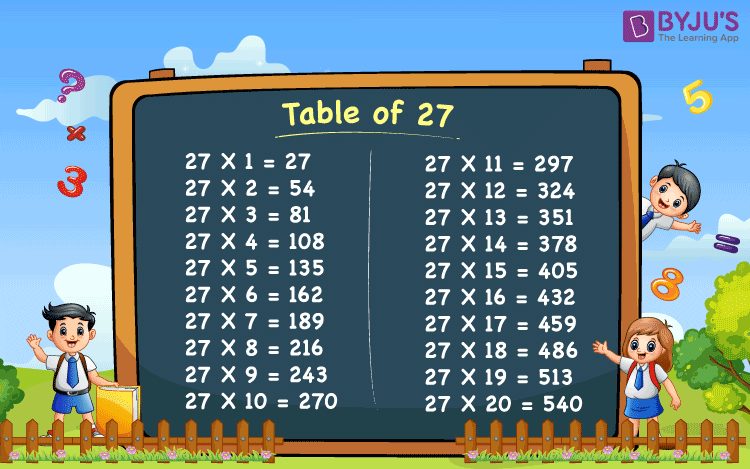Checkout JEE MAINS 2022 Question Paper Analysis : Checkout JEE MAINS 2022 Question Paper Analysis :

# Table of 27

Table of 27 is the 27 times table that includes the multiples of 27. To write a table of 27, we have to multiply 27 by a set of consecutive natural numbers. When 27 is multiplied by a natural number, then it is repeatedly added the number of times, equal to the natural number. For example, 27 x 3 = 27 + 27 + 27 = 81.

Table of 27 is provided here. Table 27 is essential for both the school students and students preparing for competitive exams like SSC, UPSC, and other national and international examinations. The multiplication table is a mathematical table used to explain the multiplication or product operation of arithmetic and algebraic systems. Students should understand that multiplication is a repeated addition process. Learning multiplication tables is a part of every child’s education.

## Table of 27 Chart## Multiplication Table of 27 (Up to 20)

Memorizing the table of 27 will help the students solve complex mathematical problems faster and easier. It is advised to memorize the multiplication table of 27 to solve more questions instead of multiplying the numbers. It saves lots of time, and you can solve the problem in a very short interval. Many students suffer in mathematics because they don’t memorize the tables. Your life will be so much easier when you remember the multiplication tables. Go through at least once when you are facing difficulties in the multiplicative table of 27.

 27 x 1 = 27 27 x 2 = 54 27 x 3 = 81 27 x 4 = 108 27 x 5 = 135 27 x 6 = 162 27 x 7 = 189 27 x 8 = 216 27 x 9 = 243 27 x 10 = 270 27 x 11 = 297 27 x 12 = 324 27 x 13 = 351 27 x 14 = 378 27 x 15 = 405 27 x 16 = 432 27 x 17 = 459 27 x 18 = 486 27 x 19 = 513 27 x 20 = 540

Students can download the PDF of the table of 27 here, to learn offline as well.

## How to read Table of 27?

• 27 x 1 = 27 ⇔Twenty-Seven times One is equal to Twenty-Seven
• 27 x 2 = 54 ⇔ Twenty-Seven times Two is equal to Fifty-Four
• 27 x 2 = 81 ⇔ Twenty-Seven times Three is equal to Eighty-One
• 27 x 2 = 108 ⇔ Twenty-Seven times Four is equal to One Hundred And Eight
• 27 x 2 = 135 ⇔ Twenty-Seven times Five is equal to One Hundred And Thirty-Five
• 27 x 2 = 162 ⇔ Twenty-Seven times Six is equal to One Hundred And Sixty-Two
• 27 x 2 = 189 ⇔ Twenty-Seven times Seven is equal to One Hundred And Eighty-Nine
• 27 x 2 = 216 ⇔ Twenty-Seven times Eight is equal to Two Hundred And Sixteen
• 27 x 2 = 243 ⇔ Twenty-Seven times Nine is equal to Two Hundred And Forty-Three
• 27 x 2 = 270 ⇔ Twenty-Seven times Ten is equal to Two Hundred And Seventy

## Solved Examples on Table of 27

Q.1: What is the value of the given expression: 27 x 5 – 10 =?

Solution: By the table of 27, we have;

27 x 5 = 135

27 x 5 – 10 = 135 – 10 = 125

Q.2: If there are 27 balls in each of the 3 baskets, how many total balls are there?

Solution: Given, Number of balls in each basket = 27

Therefore, total number of balls = 27 x 3 = 81

## Practice Questions on Table of 27

1. What is the value of 27 times 6 minus 27 times 2?
2. 27 times 8 is twice of 27 times 4. Is this statement true or false?

## Frequently Asked Questions – FAQs

### What is a table of 27?

Table of 27 is a multiplication table or 27 times table, which include multiples of 27, when multiplied by the consecutive natural numbers.

### What should be multiplied by 27 to get 108?

27 times 4 is equal to 108. Thus, 4 should be multiplied by 27.

### What are 27 times 20?

27 times 20 is equal to 540.

### What is 27 multiplied by 9?

27 multiplied by 9 is equal to 243.

Keep visiting BYJU’S – The Learning App and check out the other maths tables for different numbers and also refer to other maths-related articles

Test your Knowledge on Table of 27